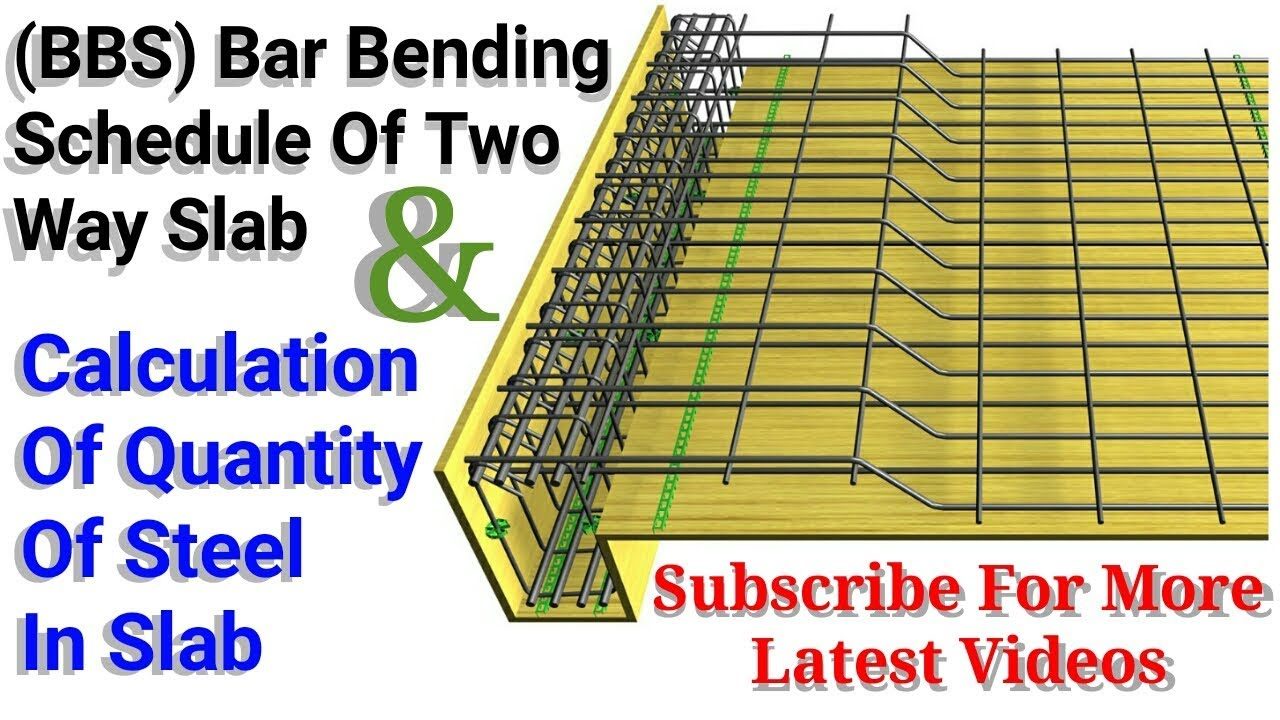HOW TO CALCULATE THE QUANTITY OF STEEL IN BEAM (BBS)

# HOW TO CALCULATE THE QUANTITY OF STEEL IN BEAM (BBS)The quantity of the beam is very easy and simple. Just you need to know about the basics of Beam. Let solve this example to understand better.
EXAMPLE
Let us we have a simply supported beam having a cross section 300×500 mm and length of the beam is 4 m. The main bars used in this beam is 20 mm and support bar diameter is 16 mm. The diameter of stirrup is 12 mm and spacing of stirrup is at an end is 100 mm and 150 mm at the mid support of the beams.
Calculate the required quantity of steel?
Cutting length of stirrups?
Calculate the weight of steel?

Continue Reading about the "HOW TO CALCULATE THE QUANTITY OF STEEL IN BEAM (BBS)" on the next page below

1.